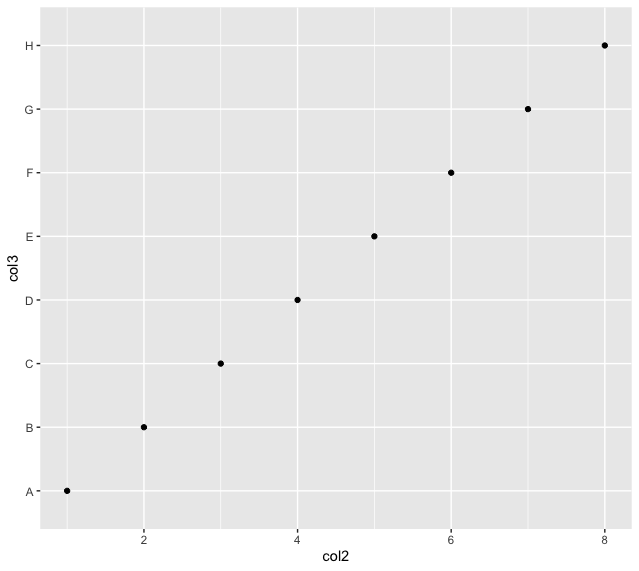Open in App
Not now

# Difference Between ggvis VS ggplot in R

• Last Updated : 02 Aug, 2022

R programming language provides many tools and packages to visualize the data points using interactive plots. The plots can be annotated or customized with different headings, titles, and color schemes to improve the readability of the data plotted.

## ggvis

The ggvis package in R is used for data visualization. It is also used to create interactive web graphics. It is also used to create interactive graphs and plots. It is an addition to the ggplot package. It provides the framework to build HTML graphs in the working space. The package can be downloaded and installed into the working space using the following command :

`install.packages("ggvis")`

## R

 `# installing the reqd library ` `library``(``"ggvis"``) ` ` `  `# creating a data frame  ` `data_frame <- ``data.frame``( ` `  ``col2 = 1:8, ` `  ``col3 = ``LETTERS``[1:8] ` `) ` `print ``(``"Data Frame"``) ` `print``(data_frame) ` ` `  `# plotting the col2 and col3 of data frame ` `layer_points``(``ggvis``(data_frame, x = ~col2, y = ~col3))`

Output

``` "Data Frame"
col2 col3
1    1    A
2    2    B
3    3    C
4    4    D
5    5    E
6    6    F
7    7    G
8    8    H```## ggplot

The ggplot2 package is a powerful and widely used package for graphic visualization. It can be used to provide a lot of aesthetic mappings to the plotted graphs. This package is widely available in R. The package can be downloaded and installed into the working space using the following command :

`install.packages("ggplot2")`

The ggplot method can be used to create a ggplot object. The graphical object is used to create plots by providing the data and its respective points. The data can be plotted using both points as well as lines.

`ggplot(data, aes = ) `

Arguments :

• data – The data to be plotted
• aes – The aesthetic mappings

## R

 `# installing the reqd library ` ` `  `library``(``"ggplot2"``) ` ` `  `# creating a data frame  ` `data_frame <- ``data.frame``( ` `  ``col2 = 1:8, ` `  ``col3 = ``LETTERS``[1:8] ` `) ` `print ``(``"Data Frame"``) ` `print``(data_frame) ` ` `  `# plotting the col2 and col3 of ` `# data frame ` `ggplot``(data_frame,``aes``(col2,col3))+ ` `  ``geom_point``()`

Output

``` "Data Frame"
col2 col3
1    1    A
2    2    B
3    3    C
4    4    D
5    5    E
6    6    F
7    7    G
8    8    H```## Table of difference between ggvis and ggplot

The following table is used to illustrate the differences between both the packages :

My Personal Notes arrow_drop_up
Related Articles